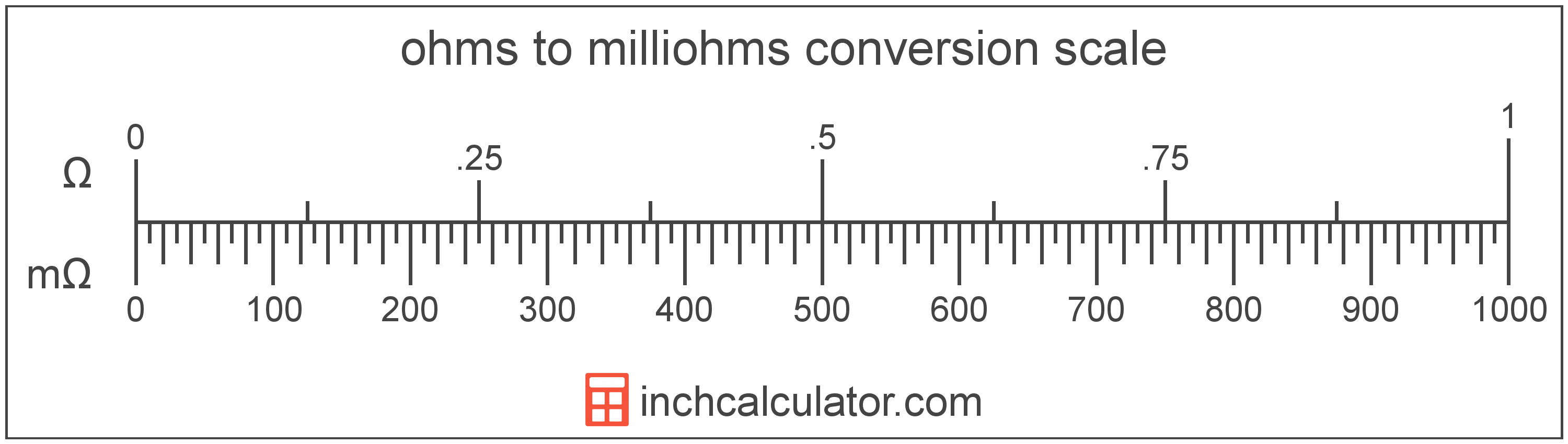# Milliohms to Ohms Converter

Enter the electrical resistance in milliohms below to get the value converted to ohms.

Results in Ohms:1 mΩ = 0.001 Ω

Do you want to convert ohms to milliohms?

## How to Convert Milliohms to Ohms

To convert a measurement in milliohms to a measurement in ohms, divide the electrical resistance by the following conversion ratio: 1,000 milliohms/ohm.

Since one ohm is equal to 1,000 milliohms, you can use this simple formula to convert:

ohms = milliohms ÷ 1,000

The electrical resistance in ohms is equal to the electrical resistance in milliohms divided by 1,000.

For example, here's how to convert 5,000 milliohms to ohms using the formula above.
ohms = (5,000 mΩ ÷ 1,000) = 5 Ω## What Is a Milliohm?

One milliohm is equal to 1/1,000 of an ohm. A milliohm is the resistance between two points of a conductor with an electrical potential difference of 1/1,000th of a volt with one ampere of current flowing through it.

The milliohm is a multiple of the ohm, which is the SI derived unit for electrical resistance. In the metric system, "milli" is the prefix for thousandths, or 10-3. Milliohms can be abbreviated as mΩ; for example, 1 milliohm can be written as 1 mΩ.

## What Is an Ohm?

An ohm is the resistance between two points of an electrical conductor transmitting a current of one ampere when the electrical potential difference is one volt between the points.

The ohm is the SI derived unit for electrical resistance in the metric system. Ohms can be abbreviated as ; for example, 1 ohm can be written as 1 Ω.

### Ohm's Law

Ohm's Law states the current flowing between two points on a conductor is proportional to the voltage and inversely proportional to the resistance. More specifically, the current is equal to the voltage divided by the resistance.

Using Ohm's Law, it's possible to express the resistance in ohms as an expression using current and voltage.

R = VV / IA

Here, the resistance R in ohms is equal to the potential difference V in volts divided by the current I in amperes.

## Milliohm to Ohm Conversion Table

Table showing various milliohm measurements converted to ohms.
Milliohms Ohms
1 mΩ 0.001 Ω
2 mΩ 0.002 Ω
3 mΩ 0.003 Ω
4 mΩ 0.004 Ω
5 mΩ 0.005 Ω
6 mΩ 0.006 Ω
7 mΩ 0.007 Ω
8 mΩ 0.008 Ω
9 mΩ 0.009 Ω
10 mΩ 0.01 Ω
20 mΩ 0.02 Ω
30 mΩ 0.03 Ω
40 mΩ 0.04 Ω
50 mΩ 0.05 Ω
60 mΩ 0.06 Ω
70 mΩ 0.07 Ω
80 mΩ 0.08 Ω
90 mΩ 0.09 Ω
100 mΩ 0.1 Ω
200 mΩ 0.2 Ω
300 mΩ 0.3 Ω
400 mΩ 0.4 Ω
500 mΩ 0.5 Ω
600 mΩ 0.6 Ω
700 mΩ 0.7 Ω
800 mΩ 0.8 Ω
900 mΩ 0.9 Ω
1,000 mΩ 1 Ω

## References

1. International Bureau of Weights and Measures, The International System of Units, 9th Edition, 2019, https://www.bipm.org/documents/20126/41483022/SI-Brochure-9-EN.pdf